### MarkPareto Call

`call MarkPareto` (result, n, objectives, minmax ) ;

The MarkPareto call identifies the Pareto-optimal set from a population of solutions. The inputs to the MarkPareto call are as follows:

 result is a one-dimensional array to accept the results of the evaluation. Its size should be the same as the size of the population being evaluated; result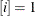if solutionis Pareto optimal, and 0 otherwise. n is a variable to receive the number of Pareto-optimal solutions. objectives is a two-dimensional array that contains the multiple objective values for each solution. It should be dimensioned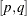, where
is the size of the population, and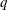is greater than or equal to the number of objectives to be considered. minmax is a one-dimensional array to specify how the objective values are to be used. It should be of size, whereis greater than or equal to the number of objectives to be considered. minmax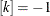if objectiveis to be minimized, minmax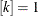if objectiveis to be maximized, minmax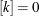if objectiveis not to be considered, and minmax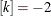designates an objective that prevents the member from being considered for Pareto optimality if it is nonzero.

The MarkPareto call is used to identify the Pareto-optimal subset from a population of solutions. See the section Optimizing Multiple Objectives for a full discussion of Pareto optimality. MarkPareto can be called from a user update routine, which is called after the individual solution objective values have been calculated and before selection takes place. To make best use of this routine, in your encoding you need to set up a segment to record all the objective values you intend to use in the Pareto-optimal set evaluation. In a user objective function, you should calculate the multiple objectives and write them to the chosen segment. In an update routine (designated with a SetUpdateRoutine call), you can use a GetSolutions call to retrieve these segments, and then pass them to a MarkPareto call. The following statements shows how this could be done:

```   subroutine update(popsize);

array objectives[1,1] /nosym;
call dynamic_array(objectives, popsize, 3);

array pareto /nosym;
call dynamic_array(pareto, popsize);

array minmax /nosym (1 -1 0);

call GetSolutions(objectives, popsize, 2);

call MarkPareto(pareto, npareto, objectives, minmax);

do i = 1 to popsize;
objectives[i,3] = pareto[i];
end;